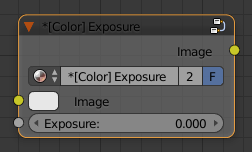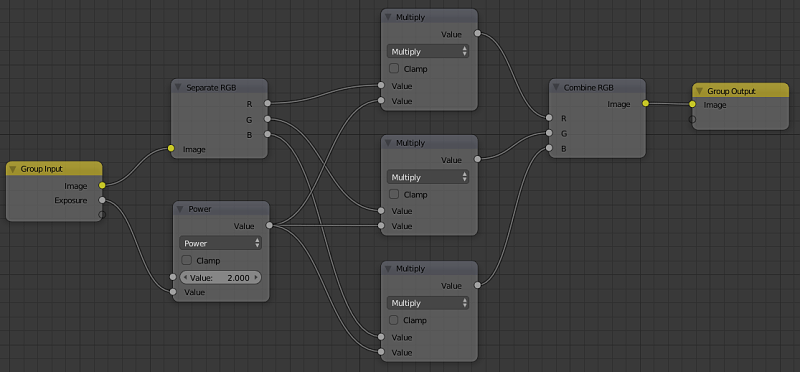# Custom Exposure Node for Blender

Exposure value (EV) is indeed essential for every photographer and computer artist. In Blender there is an exposure slider in Color Management allowing us to edit the overall exposure (in EV stops) of the output viewport or render. However, what if we wanted to edit exposure of certain material map in the node editor? Such node is still missing in Blender (2.78), but we can easily create a custom group node for that purpose.Custom exposure node for Blender.

Basically, changing exposure value in RGB color space just means multiplication of each color component by a given factor. Exposure value is defined on a 2-base logarithmic scale, so the multiplication factor should be obtained as $2^{EV}$, so

\begin{equation*} x_{\textrm{EV}} = x\ 2^{\textrm{EV}} \quad \textrm{for} \quad x \in \{R,G,B\}. \end{equation*}

We can implement this simple formula for both material and compositor node editor as follows...Node setup for the custom exposure node.